Courses

# Short Answers - Number System Class 9 Notes | EduRev

## Class 9 : Short Answers - Number System Class 9 Notes | EduRev

The document Short Answers - Number System Class 9 Notes | EduRev is a part of the Class 9 Course Class 9 Mathematics by VP Classes.
All you need of Class 9 at this link: Class 9

Q1. Find a rational number between 1 and 2.

Let x = 1 and y = 2, then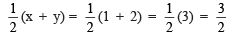Thus,  3/2 is a rational number between 1 and 2.

Q2. Write a rational number equivalent to 5/9 such that its numerator is 25.

∵ 25/5 = 5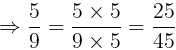Thus, 25/45 is the required rational number whose numerator is 25.

Q3. Find two rational numbers between 0.1 and 0.3.

Let x = 0.1, y = 0.3 and n = 2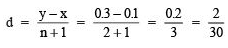∴ Two rational numbers between 0.1 and 0.3 are: x + d and x + 2d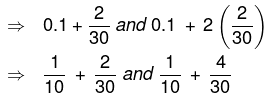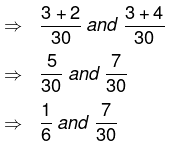Q4. Express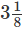in the form of a decimal.

We have,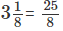Now, dividing 25 by 8,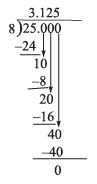Since, the remainder is 0.
∴ The process of division terminates.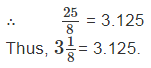Q5. Express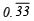as a rational number.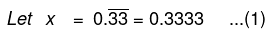Multiplying (1) by 100, we have 100x = 100 x 0.3333…
⇒ 100x = 33.3333              …(2)
Subtracting (2) from (1), we have
100x – x = 33.3333… – 0.3333…
⇒ 99x = 33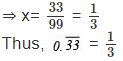Q6. Simplify: (4+ √3) (4 −3)

∵ (a + b)(a – b) = a2 – b2
(4 +√3) (4 −√3) = (4)2 – ( √3)2 = 16 – 3 = 13
Thus, (4 +√3) (4 −√3) = 13

Q7. Simplify: (√3 +√2)2

∵ (a + b)2 = a+ 2ab + b2
(√3 +√2)2 = (√3)2 + √2 ( √3 ×2) + (√2)2 = 3 + 2 √6 + 2 = 5 + 2 √6
Thus, (√3 +(√2)2 = 5 + 2√6

Q8. Rationalise the denominator of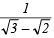Since RF of (√x - √y ) is (√x +√y )
∴ RF of (√3 - √2 ) is (√3 +√2 )
Now, we have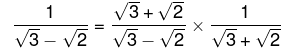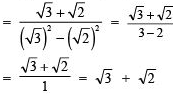Thus,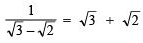Q9. Find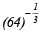As, 64 = 4 x 4 x 4 = 43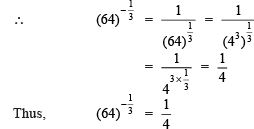Q10. Define a non-terminating decimal and repeating decimals.

The decimal expansion of some rational numbers do not have a finite number of decimal places in their decimal parts, rather they have a repeating block of digits in decimal parts. Such decimal expansion is called non-terminating and repeating decimal.
Example: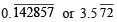Q11. What is the difference between "pure recurring decimals" and "mixed-recurring decimals"?

A decimal in which all the digits after the decimal point are repeated is called a pure recurring decimal. A decimal in which at least one of the digits after the decimal point is not repeated and then some digit(s) repeated is called a mixed recurring decimal.
Example: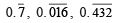are pure recurring decimals.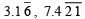are mixed recurring decimals.

Q12. What type of decimal expansion does an irrational number have?

The decimal expansion of an irrational number is "non-terminating and non-recurring."

Q13. Find a rational number lying between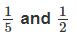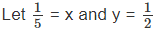Obviously x < y
A rational number lying between x and y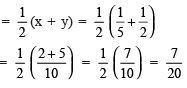Hence, 7/20  is a rational number lying between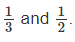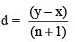∴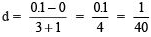∴ Three required numbers between 0 and 0.1 are: (x + d), (x + 2d) and (x + 3d)
Now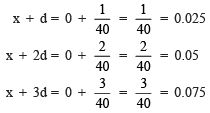Thus, three rational numbers between 0 and 0.1 are: 0.025, 0.050 and 0.075.

Q14. Express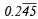as a fraction in the simplest form.

Let X == 0.24545     ... (1)
Then, multiplying (1) by 10,
We have 10X = 10 x 0.24545...
⇒ 10x = 2.4545                      ... (2)
Again multiplying (1) by 1000,
we get 1000 x X = 0.24545... x 1000
⇒ 1000X = 245.4545                ... (3)
Subtracting (2) from (3),
1000X – 10X = 245.4545... – 2.4545...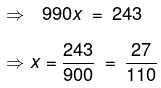Thus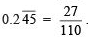Q15. If x = (2 +√5) , find the value of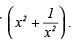We have x = 2 + √5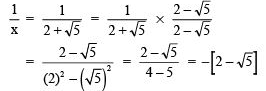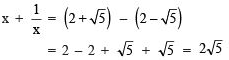Now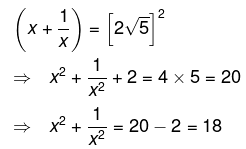Offer running on EduRev: Apply code STAYHOME200 to get INR 200 off on our premium plan EduRev Infinity!

125 docs

,

,

,

,

,

,

,

,

,

,

,

,

,

,

,

,

,

,

,

,

,

;